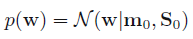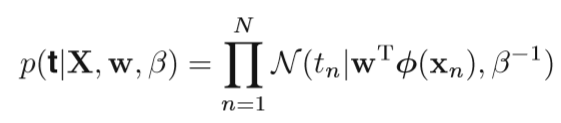# What makes this equation “exponential of quadratic”, and how is this distribution derived from it?

I am studying machine learning and I am confused by one of the derivations in our textbook. I have two questions:

1. what makes equation 3.10 a "exponential of a quadratic function of w"?
2. How is the distribution of p(w) derived here?

Here is the relevant excerpt from my textbook:

We begin our discussion of the Bayesian treatment of linear regression by introducing a prior probability distribution over the model parameters w. [...] First note that the likelihood function p(t|w) defined by (3.10) is the exponential of a quadratic function of w. The corresponding conjugate prior is therefore given by a Gaussian distribution of the form(equation 3.10):• What does the notation "$\mathcal{N}$" mean to you? Do you have a mathematical definition? – whuber Aug 9 '16 at 15:44
• Sorry, it is the normal distribution: (1/sqrt(2pi * sigma^2))*e^-(x-mu)^2/(2sigma^2)). Or at least, the multivariate version of that. – user56834 Aug 9 '16 at 16:05
• Exactly: doesn't that look like an exponential of a quadratic function of $\mu$? – whuber Aug 10 '16 at 14:55

So look at that normal density you mention in comments, for an univariate $w$:

$p(w) =\frac{1}{\sqrt{2\pi} s_0}\cdot e^{-\frac{1}{2s_0^2}(w-m_0)^2}$

Pulling the constant into the exponent:

$p(w) =e^{c_0-(w-m_0)^2/(2s_0^2)}=e^{q_0(w)}$

where $c_0 = \frac12 \log(2\pi s_0^2)$

so now it's an exponentiation of some function $q_0$

But what's $q_0$? It's a quadratic in $w$:

$q_0(w) = c_0-(w-m_0)^2/(2s_0^2) = a w^2 + bw + c$

(I'll leave you to expand it out and see what $a,b,$ and $c$ are).

The multivariate version follows the same kind of reasoning - if you write it out, in the exponent you have a quadratic form in $\mathbf{w}$.